### Joy of Problem Solving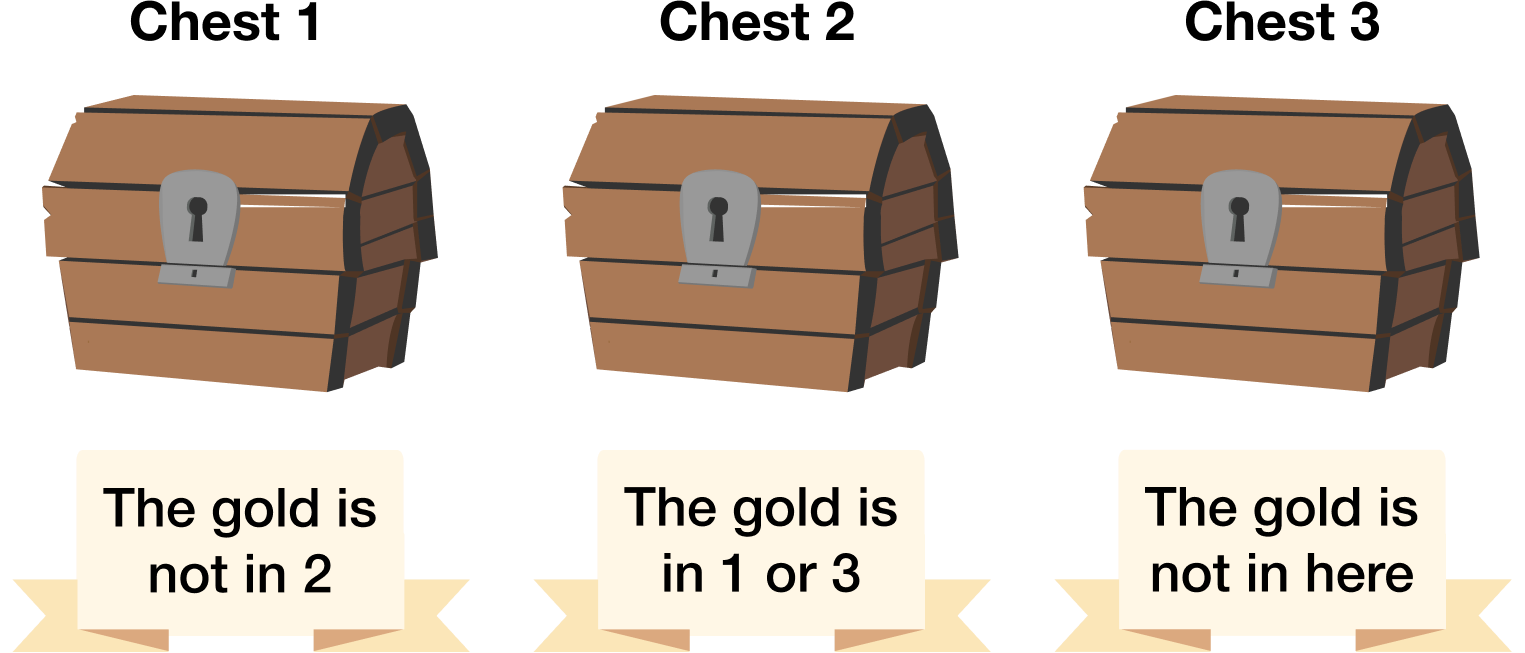If all of the statements are true, where is the gold?

# Logical Reasoning

There is gold in only one of the chests. If only one of the messages is true (and the other two are lies), where is the gold?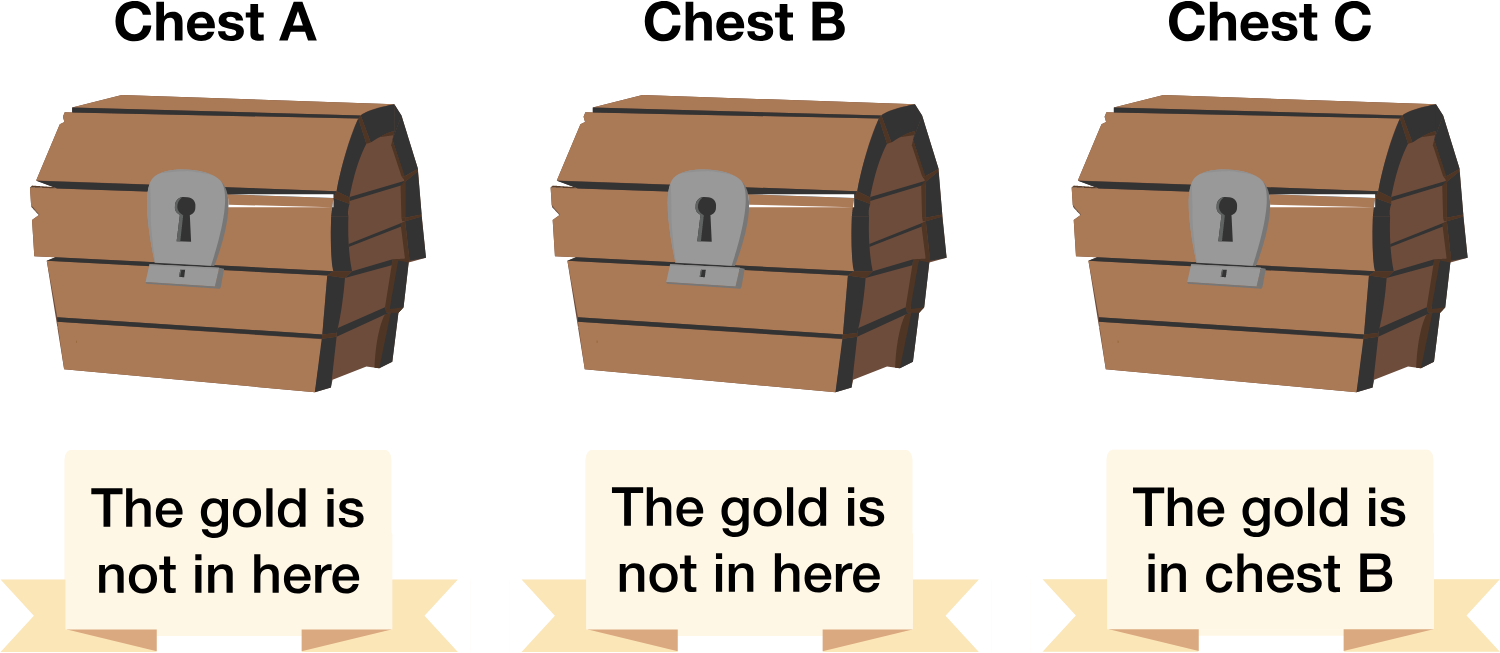# Logical Reasoning

In the previous two problems, we were given imperfect information and had to come up with creative ways to use the information given to solve the problem.

In this chapter, we explore the idea through many math problems that require this mix of creativity and logic.

# Logical Reasoning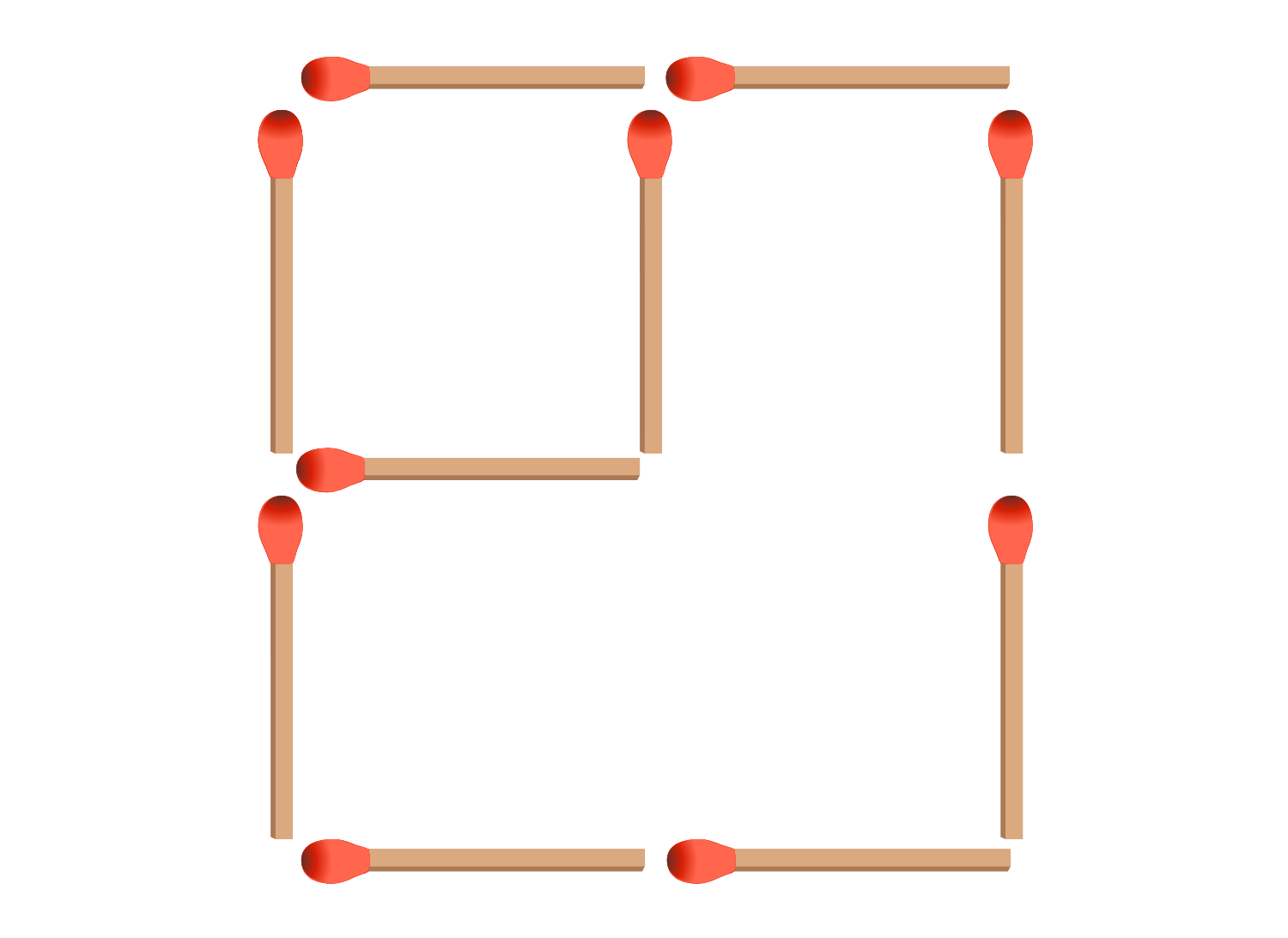In the above image, there are $2$ squares of different sizes.

What is the minimum number of matchsticks that we have to move in order to end up with exactly $3$ squares?

Note: The squares may or may not be of different sizes.

# Logical Reasoning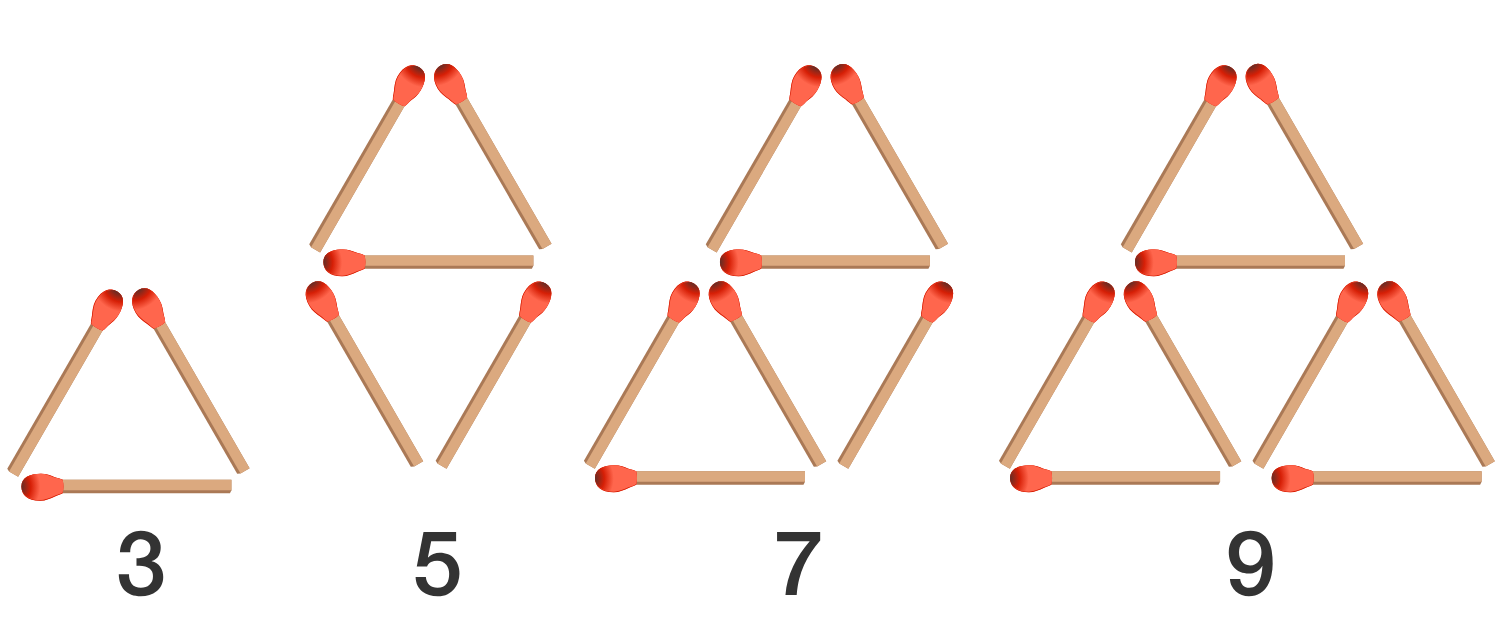From the above diagram, we see that, in order to create $1, 2, 3,$ and $4$ congruent unit equilateral triangles on a flat plane, we need $3, 5, 7,$ and $9$ matchsticks, respectively.

What is the minimum number of matchsticks on a flat plane needed to create $7$ congruent unit equilateral triangles? (Be careful! This one is trickier than it initially looks.)

# Logical Reasoning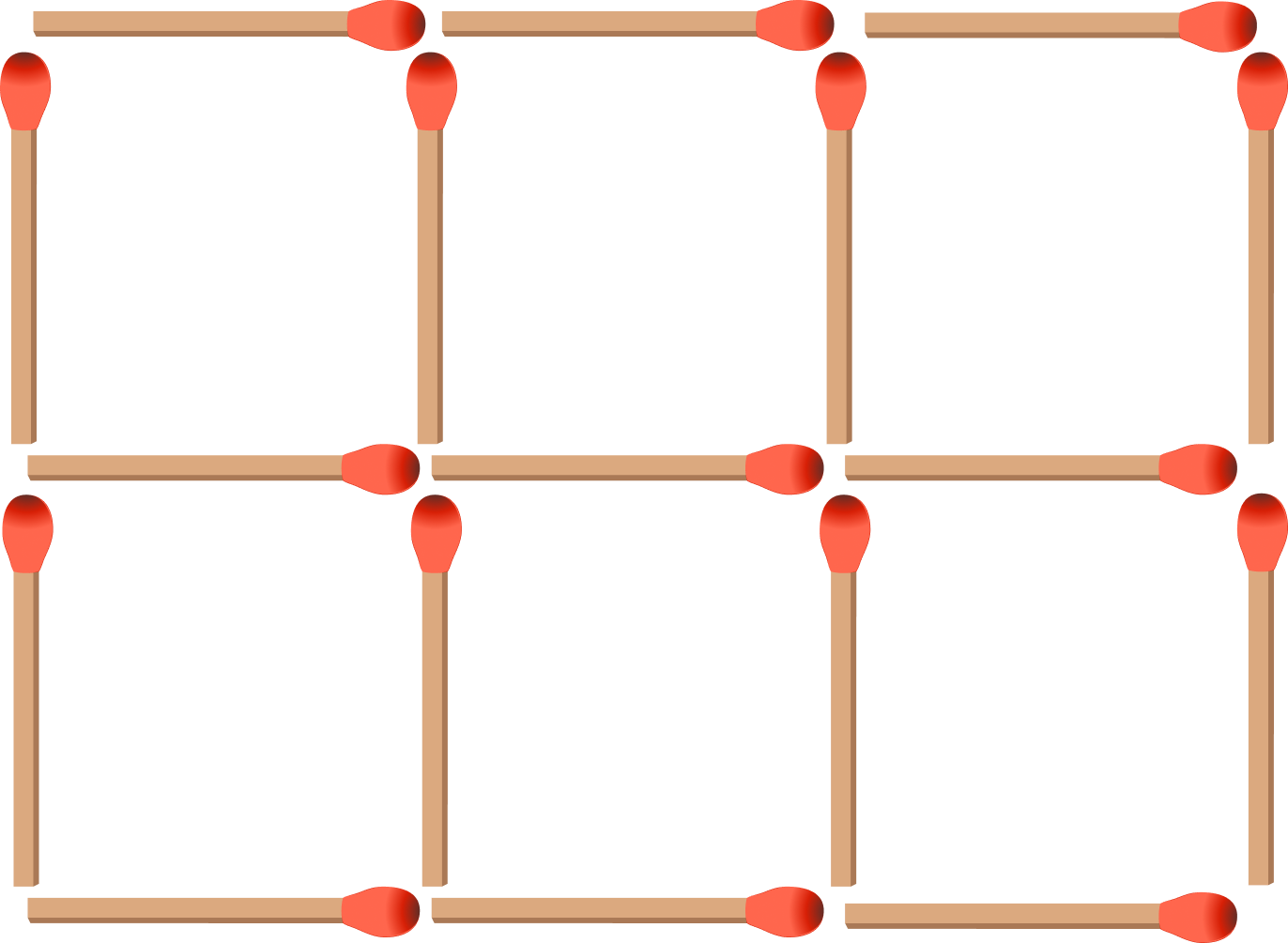What is the minimum number of matchsticks that can be removed to leave only three non-overlapping squares of the same size? (All remaining matches should be part of a square.)

Remember: Every remaining matchstick must be part of at least one of the three squares.

×